Miscellaneous

Chapter 6 Class 12 Application of Derivatives
Serial order wise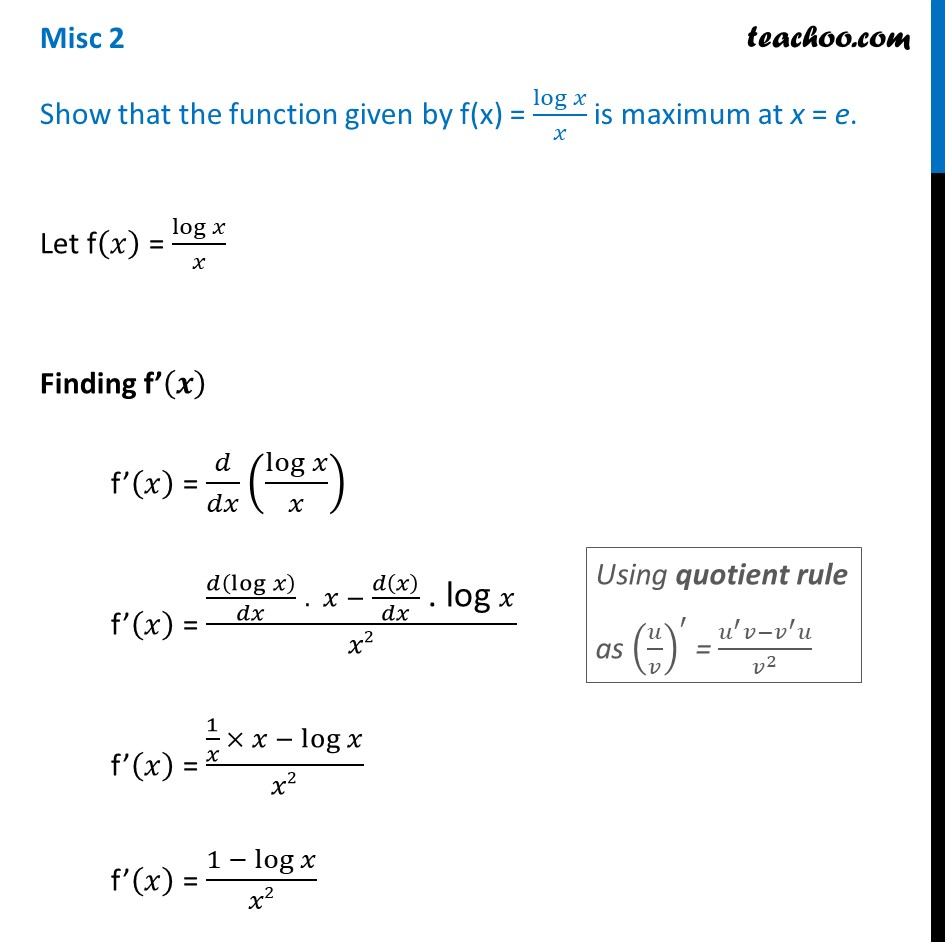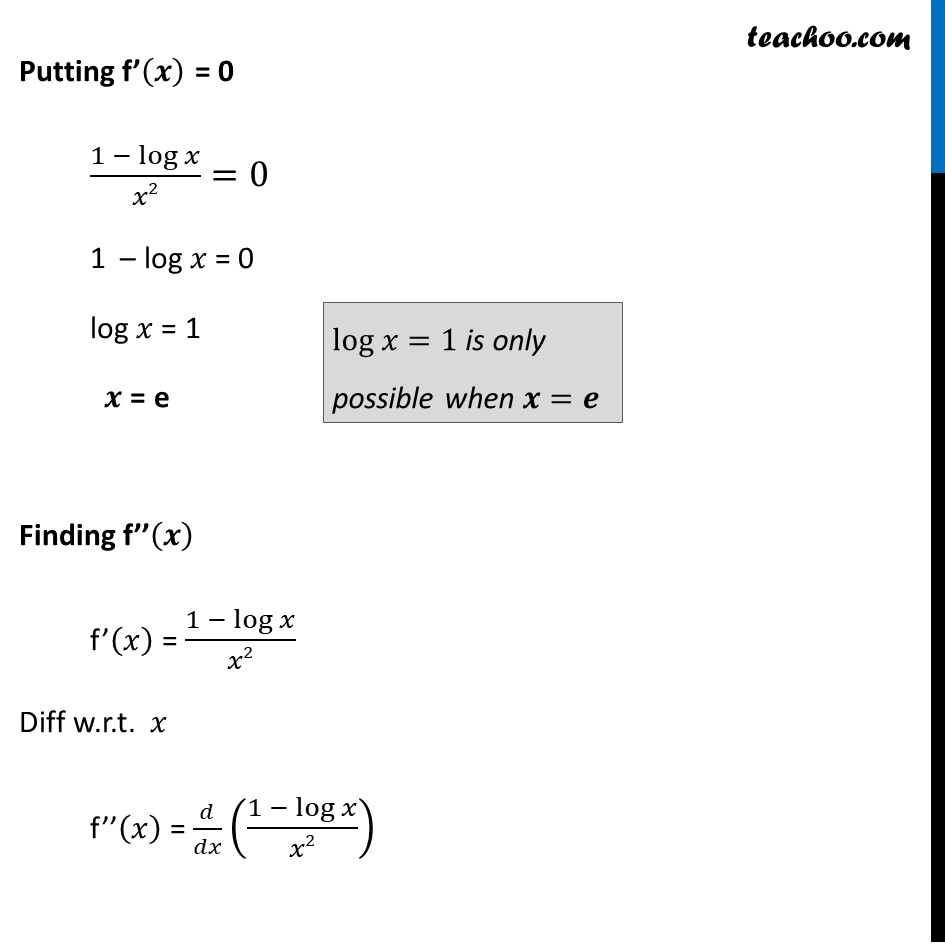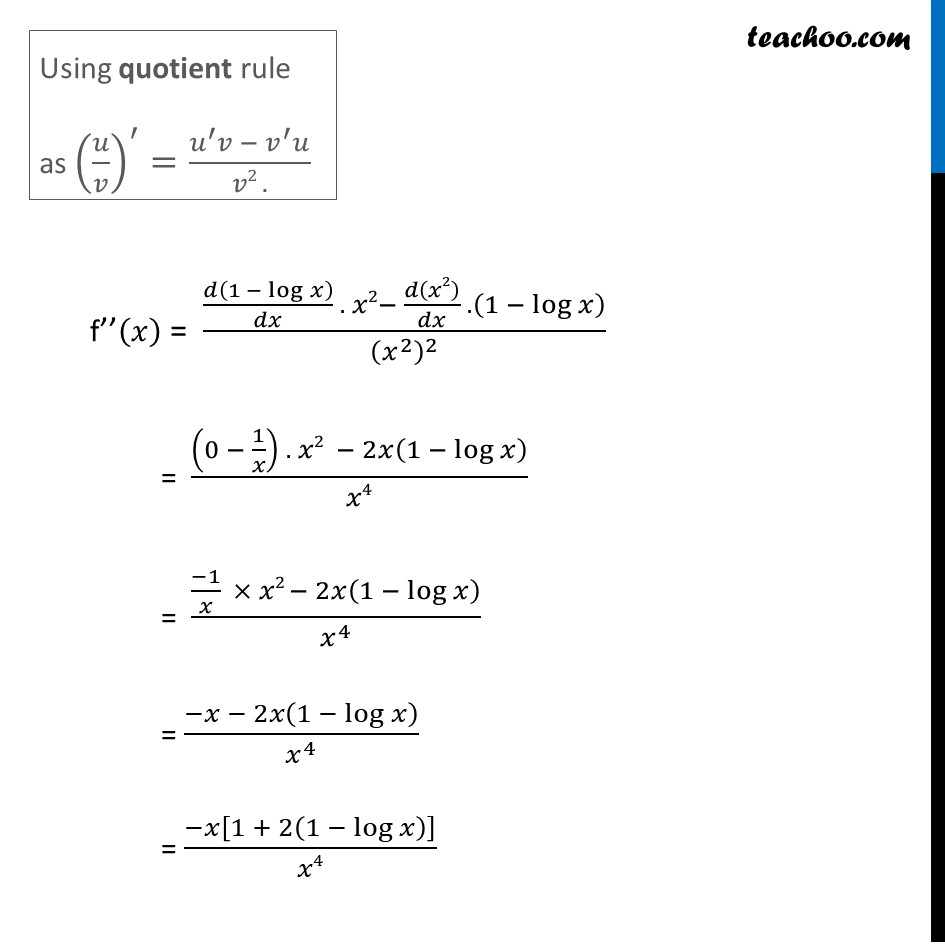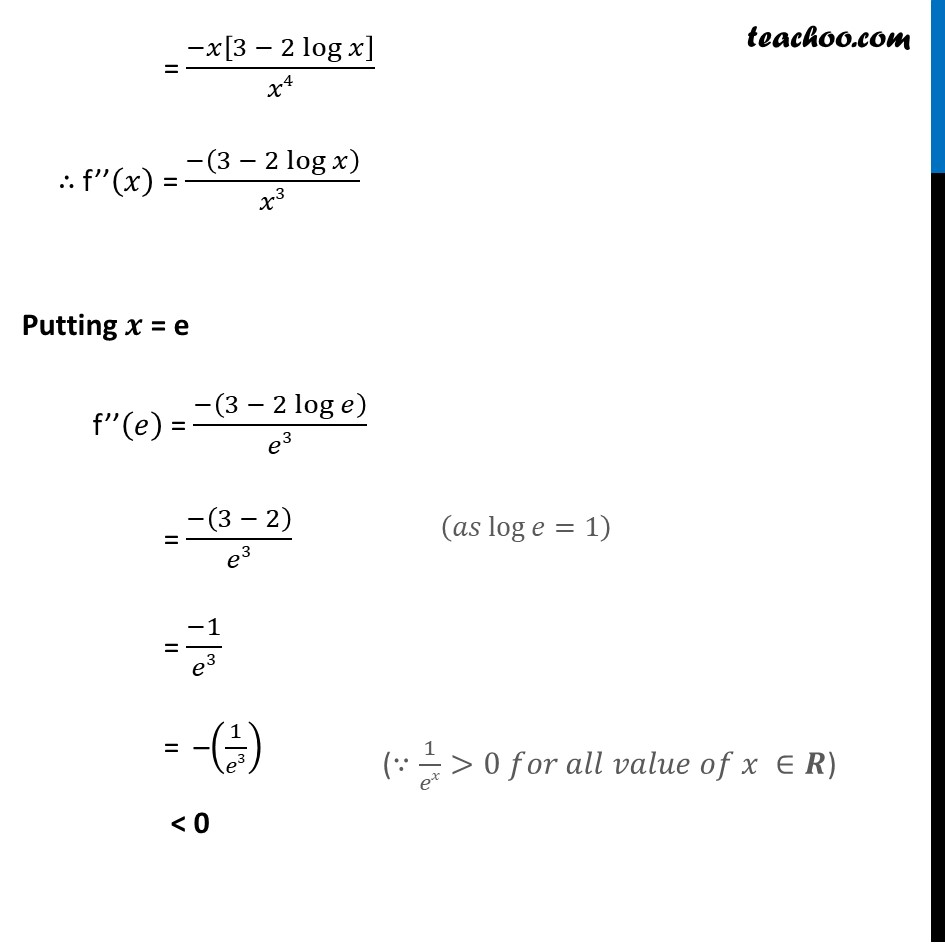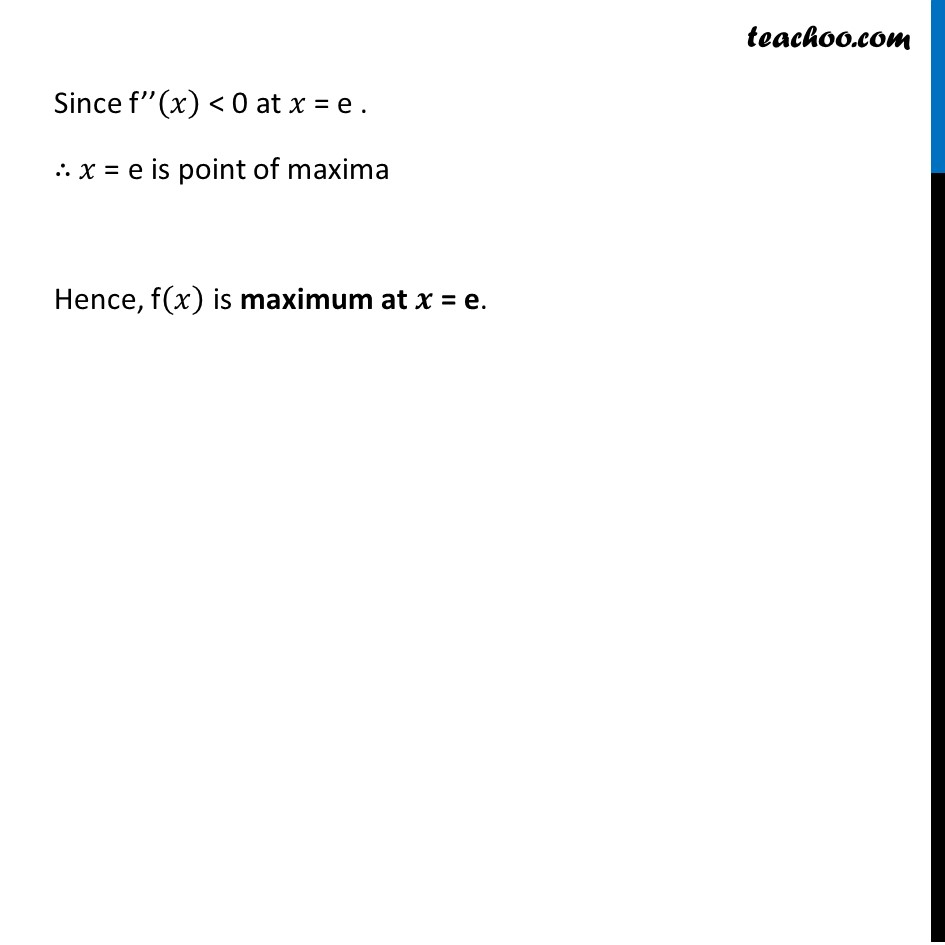Learn in your speed, with individual attention - Teachoo Maths 1-on-1 Class

### Transcript

Misc 1 Show that the function given by f(x) = log⁡𝑥/𝑥 is maximum at x = e.Let f(𝑥) = log⁡𝑥/𝑥 Finding f’(𝒙) f’(𝑥) = 𝑑/𝑑𝑥 (log⁡𝑥/𝑥) f’(𝑥) = (𝑑(log⁡𝑥 )/𝑑𝑥 " " . 𝑥 − 𝑑(𝑥)/𝑑𝑥 " . log " 𝑥)/𝑥2 f’(𝑥) = (1/𝑥 × 𝑥 − log⁡𝑥)/𝑥2 f’(𝑥) = (1 − log⁡𝑥)/𝑥2 Using quotient rule as (𝑢/𝑣)^′ = (𝑢^′ 𝑣−𝑣^′ 𝑢)/𝑣^2 Putting f’(𝒙) = 0 (1 − log⁡𝑥)/𝑥2=0 1 – log 𝑥 = 0 log 𝑥 = 1 𝒙 = e Finding f’’(𝒙) f’(𝑥) = (1 − log⁡𝑥)/𝑥2 Diff w.r.t. 𝑥 f’’(𝑥) = 𝑑/𝑑𝑥 ((1 − log⁡𝑥)/𝑥2) log 𝑥=1 is only possible when 𝒙=𝒆 Using quotient rule as (𝑢/𝑣)^′=(𝑢^′ 𝑣 − 𝑣^′ 𝑢)/(𝑣2 .) f’’(𝑥) = (𝑑(1 − log⁡𝑥 )/𝑑𝑥 . 𝑥2− 𝑑(𝑥2)/𝑑𝑥 . (1 − log⁡𝑥 ))/(𝑥^2 )^2 = ((0 − 1/𝑥) . 𝑥2 − 2𝑥(1 − log⁡𝑥 ))/𝑥4 = ((−1)/𝑥 × 𝑥2 − 2𝑥(1 − log⁡𝑥 ))/𝑥^4 = (−𝑥 − 2𝑥(1 − log⁡𝑥 ))/𝑥^4 = (−𝑥[1 + 2(1 − log⁡𝑥 )])/𝑥4 = (−𝑥[3 − 2 log⁡𝑥 ])/𝑥4 ∴ f’’(𝑥) = (−(3 − 2 log⁡𝑥 ))/𝑥3 Putting 𝒙 = e f’’(𝑒) = (−(3 − 2 log⁡𝑒 ))/𝑒3 = (−(3 − 2))/𝑒3 = (−1)/𝑒3 = –(1/𝑒3) < 0 (∵1/𝑒𝑥>0 𝑓𝑜𝑟 𝑎𝑙𝑙 𝑣𝑎𝑙𝑢𝑒 𝑜𝑓 𝑥 ∈𝑹) Since f’’(𝑥) < 0 at 𝑥 = e . ∴ 𝑥 = e is point of maxima Hence, f(𝑥) is maximum at 𝒙 = e.